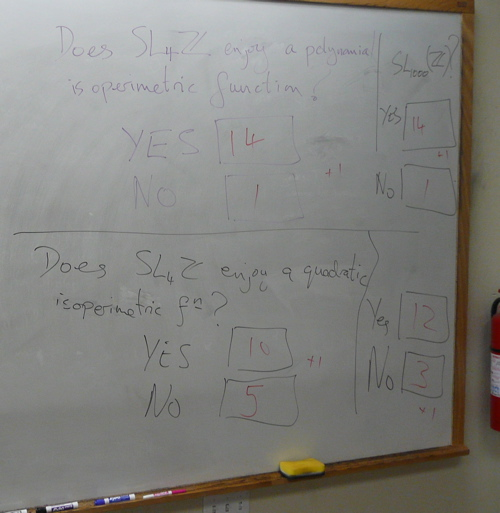The Isoperimetric Inequality for SL(n,Z)

 Update, June 2011 — R. Young, The Dehn function of SL(n;Z) — M. Bestvina, A. Eskin and K. Wortman, Filling boundaries of coarse manifolds in semisimple and solvable arithmetic groups

This page concerns a workshop held at the American Institute of Mathematics, September 8-12, 2008. Pages dedicated to the workshop on the AIM web site are here and here.

The workshop's focus was the question: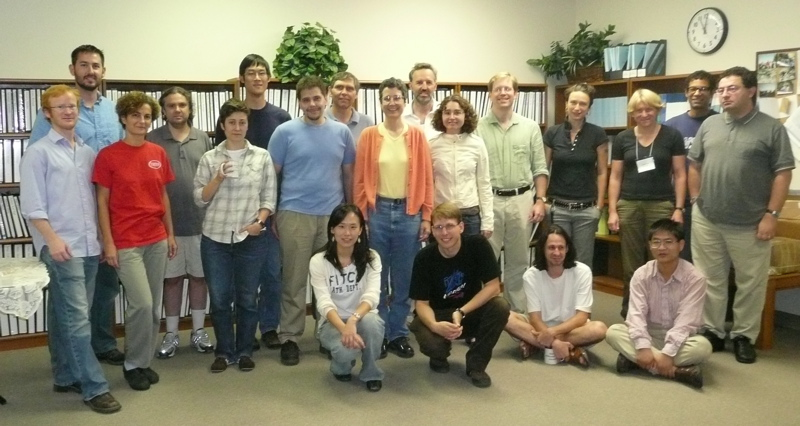Background

Isoperimetric inequalities

There are two major viewpoints:

• Combinatorial. An isoperimetric inequality for a finitely presented group is a measure of the complexity of its word problem. It concerns proving that words represent the identity by direct applications of the defining relations.

• Geometric. An isoperimetric inequality records the minimal areas of discs spanning loops in a space. The classical example is the quadratic isoperimeric inequality enjoyed by Euclidean space: loops of length L can be filled with discs of area at most a constant times the square of L.

If a group acts on a space suitably nicely, then any combinatorial isoperimetric inequality for the group is also (qualitatively) a geometric isoperimetric inequality for the space, and vice versa.

It is striking that isoperimetric inequalities remain ill-understood for many groups that are of fundamental interest in mathematics. The outstanding instance is SL(n,Z).

 What is known about isoperimetric functions for SL(n,Z)? SL(2,Z) has a linear isoperimetric inequality. In other words, SL(2,Z) is a hyperbolic group. Epstein and Thurston  proved that any isoperimetric inequality for SL(3,Z) grows at least exponentially quickly — that is, the number of relations required to reduce words w that represent the identity in SL(3,Z) to the empty word is sometimes at least exponential in the length of w. This is sharp: SL(n,Z) enjoys an exponential isoperimetric inequality for all n — a proof-strategy outlined by Gromov [12, §5A7] was taken up by Leuzinger [14, Cor. 5.4].The origins of the problem

Thurston asserted (see ) that SL(4,Z) satisfies a quadratic isoperimetric inequality (the “smallest” inequality possible for a group that is not hyperbolic). But a proof was not forthcoming, and despite great interest from the field, no-one has been able to supply one. Indeed, when n ≥ 4, it is unknown whether SL(n,Z) admits a polynomial isoperimetric function.

Thurston's assertion was reinforced and extended by Gromov [12, §5D(5)(c)] when he suggested that in SL(n,Z), higher isoperimetric inequalities concerning filling k-spheres by (k+1)-discs agree with those for Euclidean space for k ≤ n-3.

Asymptotic cones

Asymptotic cones are limits of metric spaces viewed from a sequence of increasingly distant vantage points. Reasons for thinking they might be useful in the study of SL(n,Z) are that their topology is related to filling invariants in the original space, and the asymptotioc cones of SL(n,Z) have special structure: they are "R-buildings". Notes written by Robert Young giving some background on asymptotic cones are here (pdf).

Further background

Further background on the problem of determining the optimal isoperimetric inequality for SL(n,Z) can be found in  and .

Possible attacks

There are two prominent views on how to understand isoperimetric inequalities for SL(n,Z). They are markedly different as one is in the spirit of the combinatorial approach to isoperimetric inequalites, and the other the geometric approach.The combintorial approach is to develop a qualitative version of a proof of finite presentability of SL(n,Z) (such as in ). One might use techniques such as are employed in proofs of the Lubotzky–Mozes–Raghunathan Theorem on word length in SL(n,Z) — see , , . Indeed, Thurston's assertion may be regarded as a higher-dimensional version of the Lubotzky–Mozes–Raghunathan Theorem as the former can be regarded as concerning finding efficient fillings of 1-spheres by 2-discs and latter efficient fillings of 0-spheres by 1-discs. The fact that for n ≥ 3, SL(n,Z) does not admit a combing in which the combing lines have at most polynomial length  may make this approach difficult.

In the geometric approach, one considers SL(n,Z) as a lattice in the symmetric space X = SL(n,R) / SO(n). The difficultly is that this lattice is not cocompact, and so the quadratic isoperimetric inequality enjoyed by X does not immediately pass to SL(n,Z). The attempted remedy is to remove certain open horoballs from X to give a space ("neutered space") on which SL(n,Z) acts cocompactly. But the removed horoballs have complicated intersections (indeed, there are competing definitions for horoballs to sift through), and it is unclear whether their removal destroys the isoperiemtric inequality. Partial results in this direction can be found in  and .

Spines for SL(n,Z) relate to both the combinatorial and geometric approaches. A spine for SL(n,Z) is a cell complex usually embedded in a locally symmetric space which is still a classifying space for SL(n,Z). Number Theorists have investigated the combinatorics of spines (see  and ) as well as retractions of locally symmetric spaces onto them. The problem of finding the optimal isoperimetric inequality for SL(n,Z) may be viewed as a question about the relationship between the 1- and 2-skeleta of a spine. Results such as those in  demonstrate some of the techniques Number Theorists have used to understand the cells of various dimensions (in this case (n-1)(n-2)/2) in spines for SL(n,Z).

Supplementary goals of the workshop

• Enhance our understanding of SL(n,Z), and more generally of the geometry of many important groups (particularly non-cocompact lattices in Lie groups).

• Many of the workshop participants are experts in various ways of looking at SL(n,Z) such as via its word metric, as a lattice (cocompact in neutered space), via buildings, and via number theory. We will seek to share and interrelate these perspectives.

• Develop new tools for establishing isoperimetric inequalities.

• Bring together and provoke interaction between experts on combinatorial group theory, arithmetic groups, differential geometry, and number theory.

• Stimulate progress on a number of related research directions.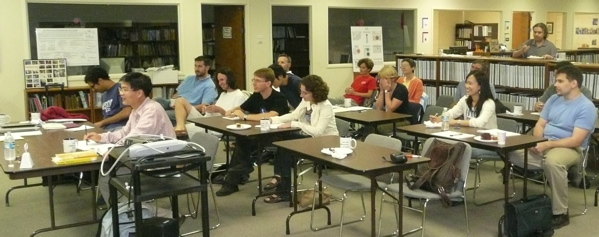Related open problems

A number of related open problems were raised before and during the workshop, including many in an Open-Problem Session led by Talia Fernós and recorded by Piotr Przytycki.

• Determine the behaviour of other filling functions for SL(n,Z) such as its isodiametric and filling length functions. Do both admit linear upper bounds? Explore connections with the distortion of IAn in Out(Fn).

• Verify Gromov's suggestion that SL(n,Z) admits a Euclidean k-th order Dehn function (concerning filling k-spheres with (k+1)-balls) for k < n-2[12, §5D(5)(c)] (cf. §2B1). Also show it is linear for all k > n-2.

More generally, is it true that any non-uniform irreducible R-rank n lattice in a semi-simple real Lie group admits a Euclidean k-th order Dehn function for all k < n-1, and is exponential for k = n-1?

For results in this direction see

• the exponential lower bound for the (n-2)-nd order Dehn function of SL(n,Z) established in ,
•  on the Q-rank one case,
• and , where an exponential first-order Dehn function is proved for R-rank two.

• Does every Q-rank one lattice in SL(n,R) or in the product of at least three copies of SL(2,R) enjoy a quadratic isoperimetric function? — cf. . Explicit examples to try are SL(2,Z[1/6]) and the Hilbert modular group SL(n,O), where O is the ring of integers of a totally real extension of Q of degree > 2.

• Prove that SL(n,R)/SO(n) with two (or three, or four,... ) intersecting horoballs (appropriately defined) enjoys a quadratic isoperimetric function.

• Determine the connectedness properties of asymptotic cones of SL(n,Z) — [12, §2B1]. Describe the horoballs one must remove from an asymptotic cone of SL(n,R)/SO(n) to get an asymptotic cone of SL(n,Z). Does every bi-Lipschitz map of an asymototic cone of SL(n,Z) to itself arise from a quasi-isometry of SL(n,Z)?

• Does SL(4,Z) enjoy Chatterji's Lδ property? — for background see  and . This condition would imply a subcubic isoperimetric function and that the asymptotic cones are simply connnected.

• Give a new elementary proof that SL(3,Z) admits an exponential isoperimetric inequality. [Gromov gave a strategy [12, §5A7], which was subsequently fleshed out by E. Leuzinger — see Corollary 5.4 in .]

• What are the higher dimensional distortion functions of SL(n,Z) ("filling at infinity")? cf. 

• What isoperimetric functions do Aut(Fn) and Out(Fn) enjoy? — , . There is a standard loop (the "baseball curve") known to require an exponential area filling in SL(3,Z) — see . It is known to lift to a loop in Out(F4) and to have a quadratic-area filling in SL(4,Z). Does this filling lift to Out(F4)?

Similar questions can be asked about Sp(2n,Z). In particular one can investigate lifting words representing the identity in Sp(2n,Z) to words in the mapping class group so as to be able use the quadratic isoperimetric inequality of the mapping class group  to establish a quadratic isoperimetric inequality for Sp(2n,Z).

• Are there analogs for finite-state automata which lend themselves to questions of filling area (e.g. automata that can "see" δ–neighborhoods of 2-disks)?

• Explicity (efficiently?) describe the geodesics in the Cayley graph of SL(n,Z) with respect to some set of generators.

• Is SL(3,Z) relatively combable as a subgroup of SL(4,Z)?

• What is the Dehn function of SL(n,Z[x]) for n > 3?

• Understand projection onto flats in SL(n,Z).

• For what k is SL(n,Fq[t]) k-connected at infinity? — one supposes k < n-2.

• Do all loops in SL(3,Z) admit quadratic (or polynomial) area fillings in SL(4,Z)? What about loops in SL(3,Z)-semidirect-Z3? In particular, what are the filling areas of the family of group constructed by Martin Kassabov (details to follow)?

• What is the abelianized (or homological) Dehn function of SL(n,Z)?

• Give a combinatorial proof of the Normal Subgroup Theorem for SL(n,Z).

• Prove any non-quasi-isometry-invariant statement on the word metric of SL(n,Z). For example, are balls in SL(n,Z) almost convex? Can there be dead ends of unbounded deapth? [The point of the non-quasi-isometry-invariant condition is to hamper use of Lubotzky-Mozes-Raghunathan.]

• Consider the following measure of hyperbolicity for a group with a word metric. Amongst all pairs of group elements x, y a distance r from the identity, take the average of d(x, y) and divide by r. [This should tend to 2 if G is a non-elementary hyperbolic group.] Is this a quasi-isometry invariant? How does it behave in SL(n,Z)?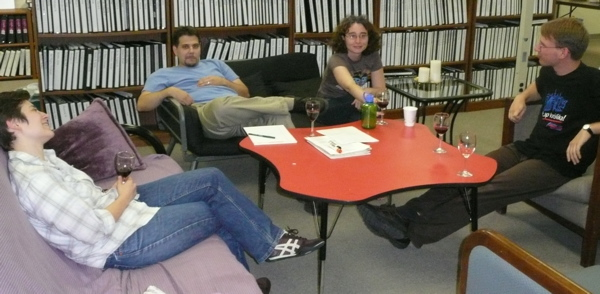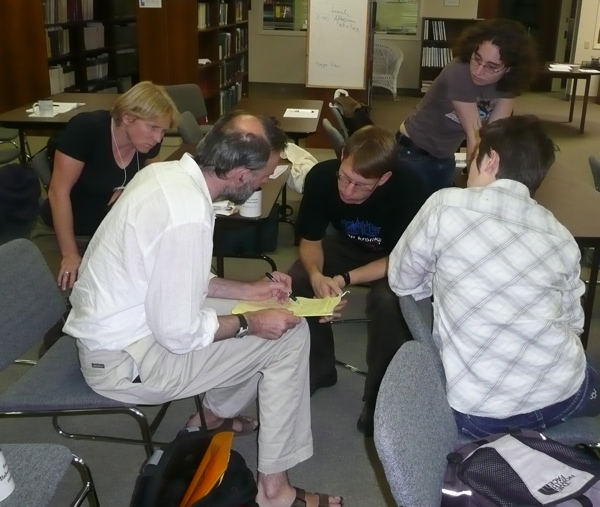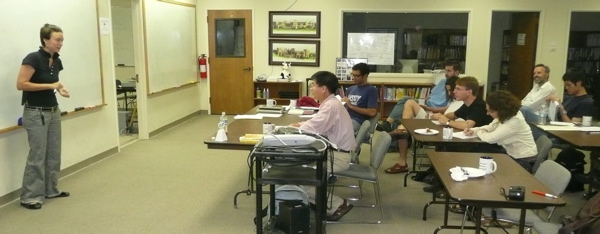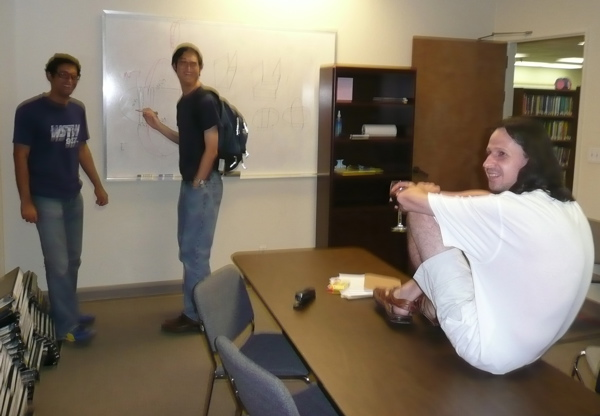Bibliography

 A. Ash, Deformation retracts with lowest possible dimension of arithmetic quotients of self-adjoint homogeneous cones, Math. Ann. 225 (1977), no. 1, 69–76 A. Ash, Small-dimensional classifying spaces for arithmetic subgroups of general linear groups, Duke Math. J. 51 (1984), no. 2, 459–468 A. Ash and L. Rudolph, The modular symbol and continued fractions in higher dimensions, Invent. Math. 55 (1979), no. 3, 241–250 M. R. Bridson and K. Vogtmann. Automorphism groups of free, surface, and free abelian groups. In Problems on Mapping Class Groups, edited by B. Farb, Proceedings of Symposia in Pure Mathematics 74, American Mathematical Society, Providence, RI, 2006, pp. 301–316. M. R. Bridson and K. Vogtmann. On the geometry of the automorphism group of a free group. Bull. London Math. Soc., 27(6): 544–552, 1995 I. Chatterji. On property (RD) for certain discrete groups. PhD Thesis, 2001, Zürich C. Drutu. Filling in solvable groups and in lattices in semisimple groups. Topology, 43:983–1033, 2004 C. Drutu, S. Mozes, M. Sapir. Divergence in lattices in semisimple Lie groups and graphs of groups. 2008 M. Elder. Lδ groups are almost convex and have sub-cubic Dehn function. Algebraic and Geometric Topology, Vol 4, 23–29, 2004. D. B. A. Epstein, J. W. Cannon, D. F. Holt, S. V. F. Levy, M. S. Paterson, and W. P. Thurston. Word Processing in Groups. Jones and Bartlett, 1992 S. M. Gersten. Isoperimetric and isodiametric functions. In G. Niblo and M. Roller, editors, Geometric group theory I, number 181 in LMS lecture notes. Camb. Univ. Press, 1993 M. Gromov. Asymptotic invariants of infinite groups.In G. Niblo and M. Roller, editors, Geometric group theory II, number 182 in LMS lecture notes. Camb. Univ. Press, 1993 A. Hatcher and K. Vogtmann. Isoperimetric inequalities for automorphism groups of free groups. Pacific J. Math., 173(2): 425–441, 1996 E. Leuzinger. On polyhedral retracts and compactifications of locally symmetric spaces. Diff Geom and its Appli., 20:293–318, 2004 E. Leuzinger and Ch. Pittet. Isoperimetric inequalities for lattices in semisimple Lie groups of rank 2. Geom. Funct. Anal., 6(3):489–511, 1996 E. Leuzinger and Ch. Pittet. On quadratic Dehn functions. Math. Z., 248(4):725–755, 2004 A. Lubotzky, S. Mozes, and M. S. Raghunathan. Cyclic subgroups of exponential growth and metrics on discrete groups. C.R. Acad. Sci. Paris, Sèrie 1, 317:723–740, 1993 A. Lubotzky, S. Mozes, and M. S. Raghunathan. The word and Riemannian metrics on lattices of semisimple groups. Inst. Hautes Études Sci. Publ. Math., 91:5–53, 2000 J. Milnor. Introduction to algebraic K-theory, volume 72 of Annals of Mathematical Studies. Princeton University Press, 1971 L. Mosher, Mapping class groups are automatic, Ann. of Math. (2) 142 (1995), no. 2, 303–384 T. R. Riley. Navigating the Cayley graphs of SLN(Z) and SLN(Fp). Geometriae Dedicata, 113(1):215–229, 2005 T. R. Riley. The Dehn function of SLn(Z), to appear in L'Enseignement Mathèmatique L. Saper, Tilings and finite energy retractions of locally symmetric spaces, Comment. Math. Helv. 72 (1997), no. 2, 167–202 K. Vogtmann. Out(Fn), Problems in Geometric Group Theory wiki K. Wortman. Lattices in Lie Groups, Problems in Geometric Group Theory wiki